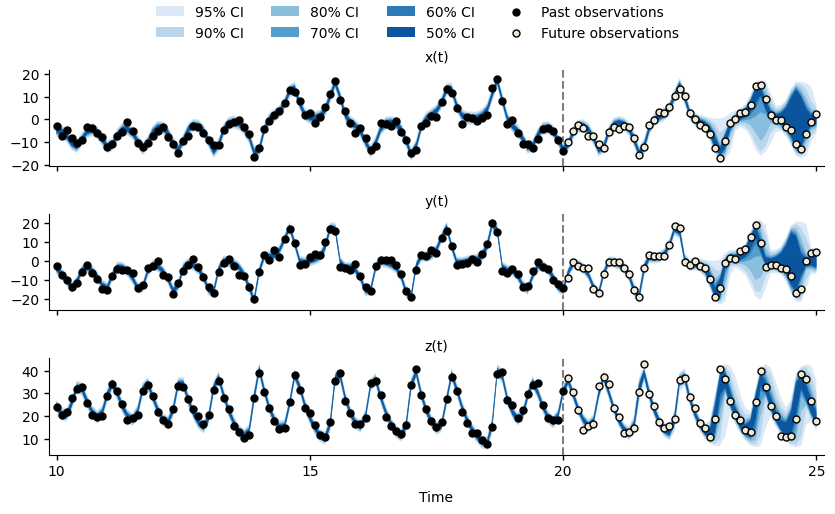# Getting Started¶

This guide assumes that you have already installed pypfilt:

# Install pypfilt with plotting support.
pip install pypfilt[plot]


See the installation instructions for further details.

This guide shows how to build a simulation model, generate simulated observations from this model, fit the model to these observations, and generate the forecasts shown below.Forecasts for $$x(t)$$, $$y(t)$$, and $$z(t)$$ of the Lorenz63 system at time $$t=20$$.¶